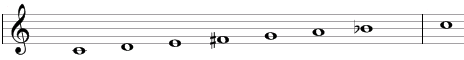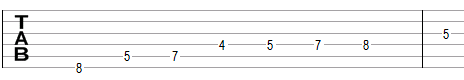# Lydian-Mixolydian scale

The Lydian-Mixolydian scale, also known as the "acoustic sale", is a secondary heptatonic scale the notes in which have the following full or half- steps in between: 1, 1, 1, ½, 1, ½, 1.

One example of the scale is C, D, E, F#, G, A, Bb. This example is shown below in traditional notation and in guitar tablature notation.This scale is called Lydian-Mixolydian as it starts similarly to the Lydian scale (the Lydian scale has the steps 1, 1, 1, ½, 1, 1, ½), but ends similarly to the Mixolydian scale (the Mixolydian scale has the steps 1, 1, ½, 1, 1, ½, 1).

This scale is also known as the "acoustic scale", because it can be approximated with a series of harmonics. Starting with an arbitrary base frequency (say C = 261.63 Hz), a computation of the acoustic scale would be:

C = 1 * 261.63 Hz
C = 2 * 261.63 = 523.25 Hz
G = 3 * 261.63 = 784.88 Hz
C = 4 * 261.63 = 1,046.5 Hz
E = 5 * 261.63 = 1,308.13 Hz

and so on computing G = 1,569.75 Hz, Bb = 1,831.38 Hz, C = 2,093 Hz, D = 2,354.63 Hz, E = 2,616.26 Hz, F# = 2,877.88 Hz, G = 3,139.51 Hz, A = 3,401.13 Hz, and Bb = 3,684.56 Hz. These last notes are the notes of the acoustic scale in C. This computation thus uses harmonics of the base frequency.

The problem with the computation above is that it is only an approximation. An equal tempered scale, for example, will compute F# = 261.63 * (2^(42 / 12)) = 2,959.96 Hz (there are 42 semitones between C = 261.63 Hz and F# = 2,959.96 Hz). This equal tempered F# is about one half of a semitone (48.6 cents) above the F# computed with the use of harmonics. A is similarly 59.4 cents lower than an equal tempered A, which makes it closer to an Ab.

## Modes of the Lydian-Mixolydian scale

The following are modes of the Lydian-Mixolydian scale.

1. Lydian-Mixolydian, or acoustic, with steps 1, 1, 1, ½, 1, ½, 1 (C, D, E, F#, G, A, Bb in the example above).
2. Minor with a raised third, or major-minor, with steps 1, 1, ½, 1, ½, 1, 1 (D, E, F#, G, A, Bb, C).
3. Locrian with a raised second, with steps 1, ½, 1, ½, 1, 1, 1 (E, F#, G, A, Bb, C, D).
4. Locrian with a flat fourth, or altered, with steps ½, 1, ½, 1, 1, 1, 1 (F#, G, A, Bb, C, D, E).
5. Melodic ascending minor, with steps 1, ½, 1, 1, 1, 1, ½ (G, A, Bb, C, D, E, F#).
6. Phrygian with a raised sixth, with steps ½, 1, 1, 1, 1, ½, 1 (A, Bb, C, D, E, F#, G).
7. Lydian with a raised fifth, with steps 1, 1, 1, 1, ½, 1, ½ (Bb, C, D, E, F#, G, A).

## Three-note chords on the Lydian-Mixolydian scale

The following are common triads built on the notes of the Lydian-Mixolydian scale.

• On the root of the scale (on the tonic): major chord (e.g., C composed of C, E, G) or suspended chord (Csus2 = C, D, G).
• On the second note (on the supertonic): major chord (D = D, F#, A), augmented chord (Daug = D, F#, Bb), or suspended chords (Dsus4 = D, G, A and Dsus2 = D, E, A).
• On the third note (on the mediant): diminished chord (Edim = E, G, Bb).
• On the fourth note (on the subdominant): augmented chord (F#aug = F#, Bb, D) or diminished chord (F#dim = F#, A, C).
• On the fifth note (on the dominant): minor chord (Gm = G, Bb, D) or suspended chords (Gsus4 = G, C, D and Gsus2 = G, A, D).
• On the sixth note (on the submediant): minor chord (Am = A, C, E) or suspended chord (Asus4 = A, D, E).
• On the seventh note (on the leading tone: augmented chord (Bbaug = Bb, D, F#).

## Four-note chords on the Lydian-Mixolydian scale

The following are seventh chords built on the notes of the Lydian-Mixolydian scale.

• On the first note: dominant seventh chord (e.g. C7 composed of C, E, G, Bb).
• On the second note: dominant seventh chord (D7 = D, F#, A, C) or augmented seventh chord (dominant seventh chord with a sharp fifth, D7#5 = D, F#, Bb, C).
• On the third note: half-diminished seventh chord (minor seventh chord with a flat fifth, Em7b5 = E, G, Bb, D).
• On the fourth note: augmented seventh chord (F#7#5 = F#, Bb, D, E) or half-diminished seventh chord (F#m7b5 = F#, A, C, E).
• On the fifth note: minor-major seventh chord (Gmmaj7 = G, Bb, D, F).
• On the sixth note: minor seventh chord (Amin7 = A, C, E, G).
• On the seventh note: augmented major seventh chord (major seventh chord with a raised fifth, Bbmaj7#5 = Bb, D, F#, A).

## Intervals on the Lydian-Mixolydian scale

The Lydian-Mixolydian scale is composed of the following intervals.

• A major second, e.g., the interval between C and D is equal to two semitones.
• A major third, e.g., the interval between C and E is equal to four semitones.
• An augmented fourth, e.g., the interval between C and F# is equal to six semitones.
• A perfect fifth, e.g., the interval between C and G is equal to seven semitones.
• A major sixth, e.g., the interval between C and A is equal to nine semitones.
• A minor seventh, e.g., the interval between C and Bb is equal to ten semitones.

Scale, Scale (index)

### Filtered HTML

• Freelinking helps you easily create HTML links. Links take the form of [[indicator:target|Title]]. By default (no indicator): Click to view a local node.